# 不为人知的 String（值得一看）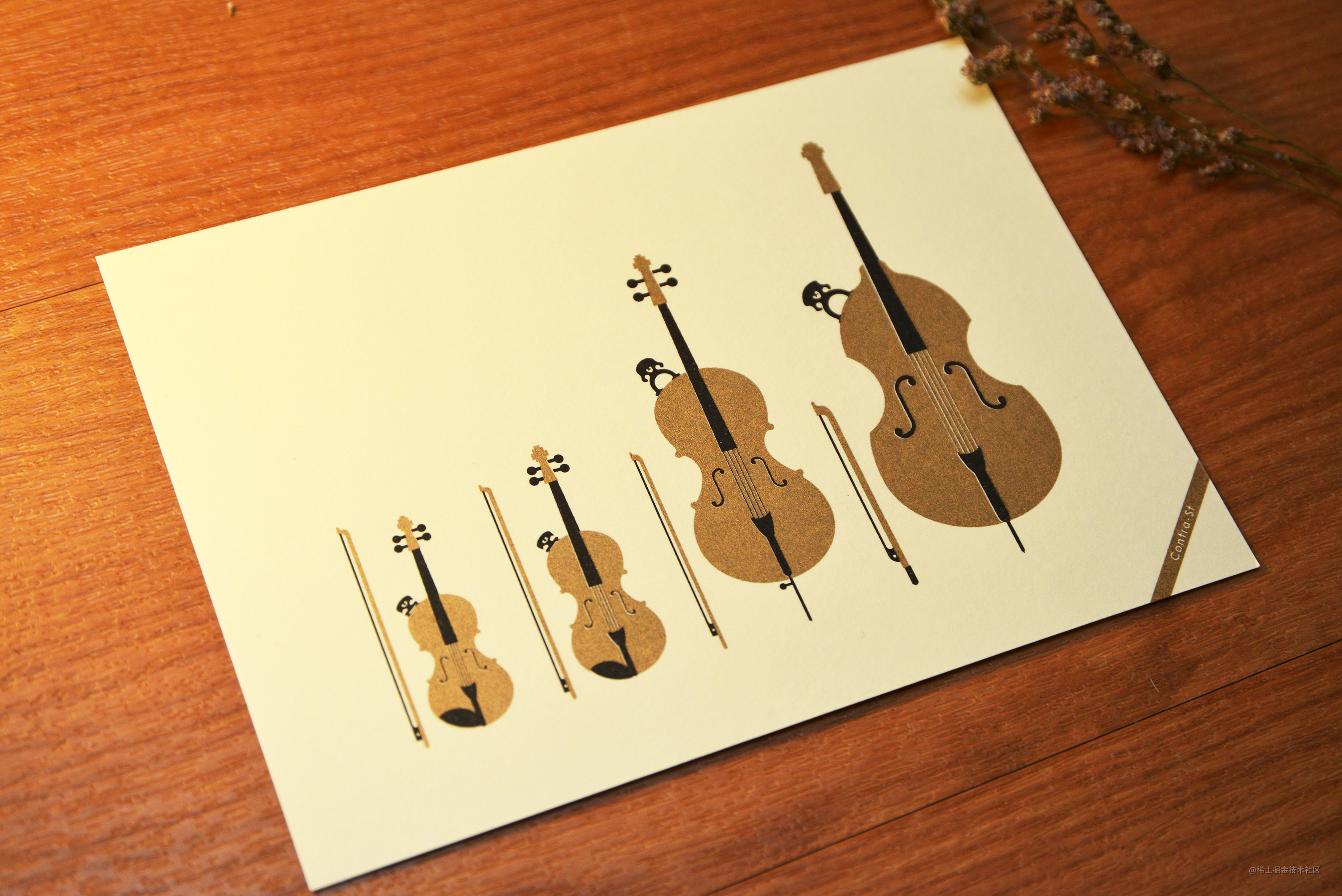## 分析源码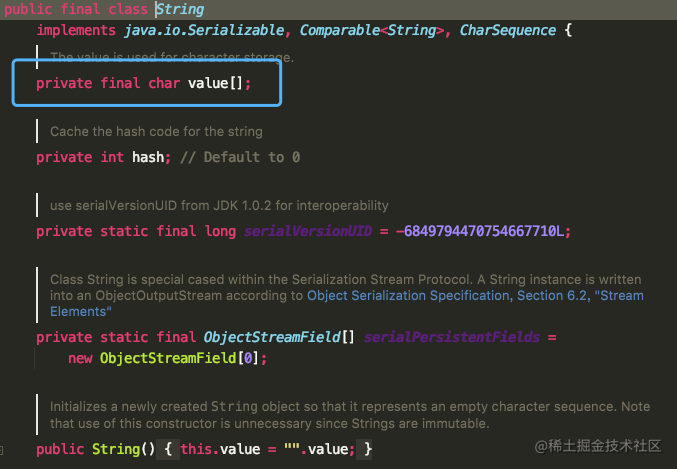``````//无参构造方法
public String() {
this.value = "".value;
}

//string为参数的构造方法
public String(String original) {
this.value = original.value;
this.hash = original.hash;
}

// char[] 为参数构造方法
public String(char value[]) {
this.value = Arrays.copyOf(value, value.length);
}

// StringBuffer 为参数的构造方法
public String(StringBuffer buffer) {
synchronized(buffer) {
this.value = Arrays.copyOf(buffer.getValue(), buffer.length());
}
}

// StringBuilder 为参数的构造方法
public String(StringBuilder builder) {
this.value = Arrays.copyOf(builder.getValue(), builder.length());
}

• equals() 比较两个字符串是否相等

• indexOf()：查询字符串首次出现的下标位置

• contains()：查询字符串中是否包含另一个字符串

• toLowerCase()：把字符串全部转换成小写

• toUpperCase()：把字符串全部转换成大写

• length()：查询字符串的长度

• trim()：去掉字符串首尾空格

• split()：把字符串分割并返回字符串数组

...........还有一些，这里不进行赘述

## equals() 比较两个字符串是否相等

``````//这是我们经常使用的方法
public boolean equals(Object anObject) {
// 对象引用相同直接返回 true
if (this == anObject) {
return true;
}
// 判断需要对比的值是否为 String 类型，如果不是则直接返回 false
if (anObject instanceof String) {
String anotherString = (String)anObject;
int n = value.length;
if (n == anotherString.value.length) {
// 把两个字符串都转换为 char 数组对比
char v1[] = value;
char v2[] = anotherString.value;
int i = 0;
// 循环比对两个字符串的每一个字符
while (n-- != 0) {
// 如果其中有一个字符不相等就 true false，否则继续对比
if (v1[i] != v2[i])
return false;
i++;
}
return true;
}
}
return false;
}

String类型重写了Object中的equals()方法，equals()方法需要传递一个Object类型的参数值，在比较时会先通过 instanceof判断是否为String类型，如果不是则会直接返回false，instanceof的使用如下：

``````Object oString = "123";
Object oInt = 123;
System.out.println(oString instanceof String); // 返回 true
System.out.println(oInt instanceof String); // 返回 false

OK 其他方法 大家可以下面自己进去看下。下面我们通过面试题去研究下

## 为什么String类型要用final修饰？（高频面试题）

Java 语言之父 James Gosling 的回答是，他会更倾向于使用 final，因为它能够缓存结果，当你在传参时不需要考虑谁会修改它的值；如果是可变类的话，则有可能需要重新拷贝出来一个新值进行传参，这样在性能上就会有一定的损失。

James Gosling 还说迫使 String 类设计成不可变的另一个原因是安全，当你在调用其他方法时，比如调用一些系统级操作指令之前，可能会有一系列校验，如果是可变类的话，可能在你校验过后，它的内部的值又被改变了，这样有可能会引起严重的系统崩溃问题，这是迫使 String 类设计成不可变类的一个重要原因。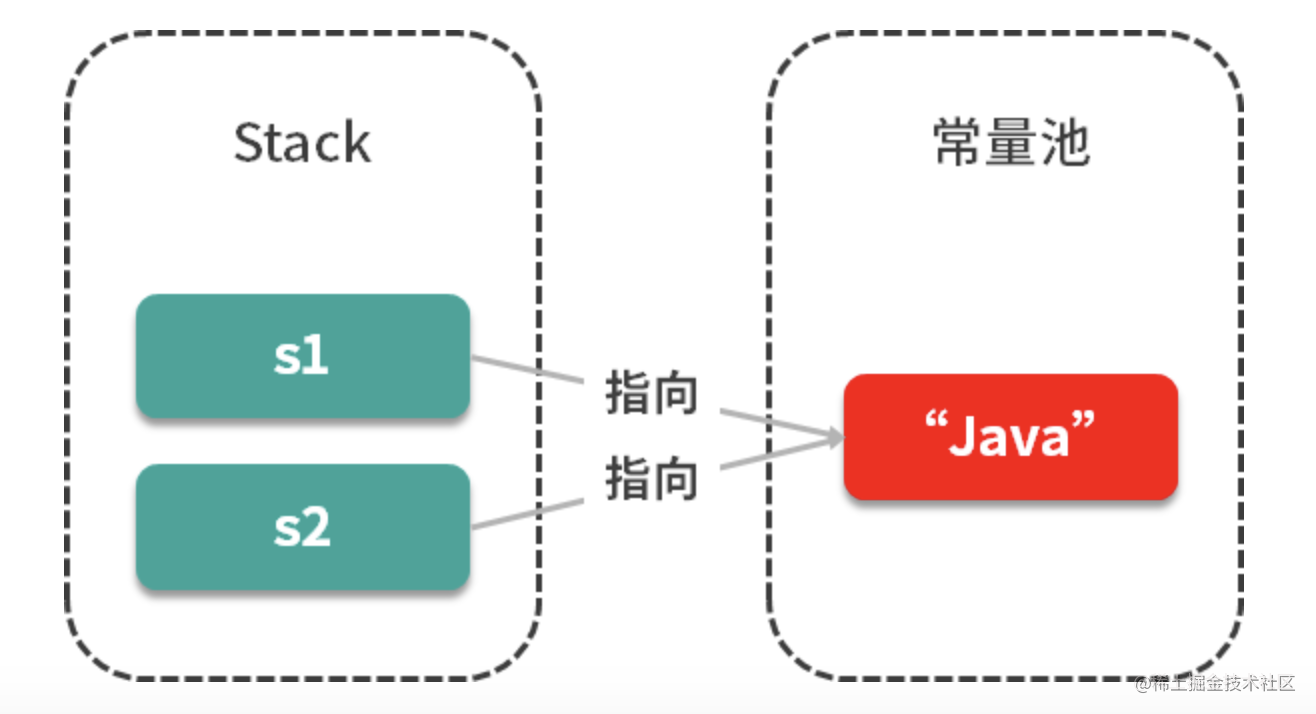## == 和 equals的区别是什么？（高频面试题）

``````public boolean equals(Object obj) {
return (this == obj);
}

public boolean equals(Object anObject) {
// 对象引用相同直接返回 true
if (this == anObject) {
return true;
}
// 判断需要对比的值是否为 String 类型，如果不是则直接返回 false
if (anObject instanceof String) {
String anotherString = (String)anObject;
int n = value.length;
if (n == anotherString.value.length) {
// 把两个字符串都转换为 char 数组对比
char v1[] = value;
char v2[] = anotherString.value;
int i = 0;
// 循环比对两个字符串的每一个字符
while (n-- != 0) {
// 如果其中有一个字符不相等就 true false，否则继续对比
if (v1[i] != v2[i])
return false;
i++;
}
return true;
}
}
return false;
}

## String 和StringBuilder、StringBuffer有什么区别？（高频面试题）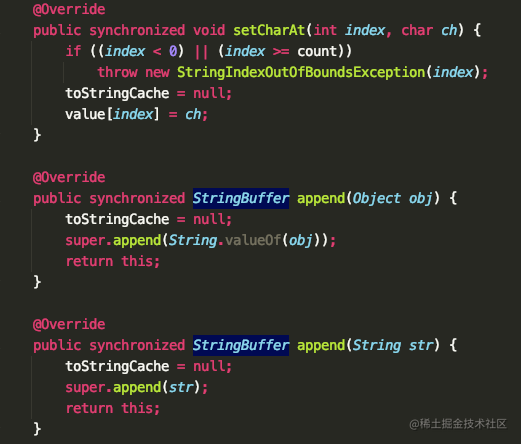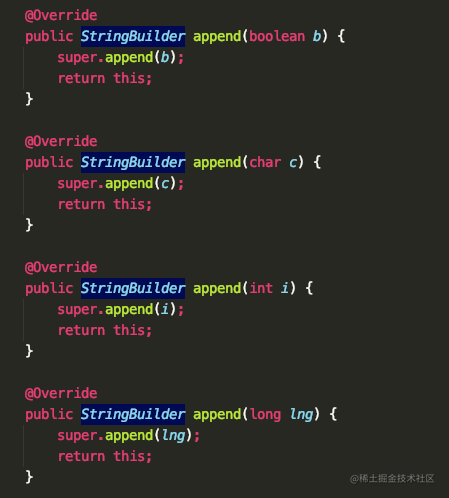OK 今天的学习就到这里。我们下期再见

copy对象，这个操作有点骚！